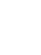# Matematički model površina - MnoženjePreuzimanje (Download)Ugradnja (Embed) zatvori Ugradi (Embed) izvršnu kopiju ove simulacije Koristi ovaj HTML za ugradnju (embed) izvršne kopije ove simulacije. Možeš mijenjati dimenzije prikaza (širinu i visinu ekrana) mijenjajući "width" i "height" atribute u HTML. Ugradi sliku. Klikom na nju pokretat će se simulacija.
Klikni i započni
Koristi ovaj HTML kod da bi se pojavio ekran sa natpisom "Klikni i započni" ("Click to Run"). Partial Products Factors Množenje PhET je podržаn od:i nаstаvnikа kаo što ste Vi!

• Partial Products
• Factors
• Množenje
• Area Model

### Description

Build rectangles of various sizes and relate multiplication to area. Discover new strategies for multiplying large numbers. Use the game screen to test your problem solving strategies!

### Primjeri obrazovnih ciljeva

• Recognize that area represents the product of two numbers and is additive.
• Represent a multiplication problem as the area of a rectangle, proportionally or using generic area.
• Develop and justify a strategy to determine the product of two multi-digit numbers by representing the product as an area or the sum of areas.

### Standards Alignment

#### Common Core - Math

3.MD.C.7
Relate area to the operations of multiplication and addition.
3.MD.C.7c
Use tiling to show in a concrete case that the area of a rectangle with whole-number side lengths a and b + c is the sum of a × b and a × c. Use area models to represent the distributive property in mathematical reasoning.
3.MD.C.7d
Recognize area as additive. Find areas of rectilinear figures by decomposing them into non-overlapping rectangles and adding the areas of the non-overlapping parts, applying this technique to solve real world problems.
4.NBT.B.5
Multiply a whole number of up to four digits by a one-digit whole number, and multiply two two-digit numbers, using strategies based on place value and the properties of operations. Illustrate and explain the calculation by using equations, rectangular arrays, and/or area models.
6.EE.A.3
Apply the properties of operations to generate equivalent expressions. For example, apply the distributive property to the expression 3 (2 + x) to produce the equivalent expression 6 + 3x; apply the distributive property to the expression 24x + 18y to produce the equivalent expression 6 (4x + 3y); apply properties of operations to y + y + y to produce the equivalent expression 3y.
6.EE.A.4
Identify when two expressions are equivalent (i.e., when the two expressions name the same number regardless of which value is substituted into them). For example, the expressions y + y + y and 3y are equivalent because they name the same number regardless of which number y stands for..
6.NS.B.4
Find the greatest common factor of two whole numbers less than or equal to 100 and the least common multiple of two whole numbers less than or equal to 12. Use the distributive property to express a sum of two whole numbers 1-100 with a common factor as a multiple of a sum of two whole numbers with no common factor. For example, express 36 + 8 as 4 (9 + 2)..
7.EE.A.1
Apply properties of operations as strategies to add, subtract, factor, and expand linear expressions with rational coefficients.
7.NS.A.2c
Apply properties of operations as strategies to multiply and divide rational numbers.
Version 1.2.1

### Teacher TipsVodič za nastavnike (pdf) sadrži savjete kreirane оd strane PhET tima. ( PDF ).

### Teacher-Submitted Activities

Middle School Math Sim AlignmentAmanda McGarry MS (SŠ) Ostalo Matematika
SECUNDARIA: Alineación PhET con programas de la SEP México (2011 y 2017)Diana López HS (VŠ)
MS (SŠ)
Ostalo Biologija
Fizika
Hemija
Matematika
PREPARATORIA: Alineación de PhET con programas de la DGB México (2017)Diana López HS (VŠ)
UG-Intro (VSŠ)
Ostalo Hemija
Matematika
Fizika
PRIMARIA: Alineación con programas de la SEP México (2011 y 2017)Diana López K-5 (OŠ)
MS (SŠ)
Guided
Discuss
Lab
Demo
DZ
Fizika
Astronomija
Matematika
Hemija
Jezik Downloador RunTips
Albanski All shqipModeli i Syprinës: Shumëzimi
Arapski All العربيةضرب مساحة نموذج
Baskijski All EuskaraAzalera-modeloan biderkaketa
Brazilski Portugalski All português (Brasil)Modelo de Área: Multiplicação
Danski All DanskArealmodel, gange
Engleski All EnglishArea Model Multiplication
Finski All suomiPinta-alamallilla kertominen
Francuski All françaisModélisation de la multiplication par les aires
Grčki All ΕλληνικάΥπολογισμός εμβαδού - Πολλαπλασιασμός
Gujarati All Gujaratiક્ષેત્રફળનું ગુણાકારનું મોડેલ - નિકુંજ સવાણી દ્વારા
Hindu All हिंदीनमूने का क्षेत्रफल - गुणन
Holandski All NederlandsOppervlakte model vermenigvuldiging
Italijanski All italianoRappresentazione grafica della moltiplicazione
Japanski All 日本語面積を求める
Kazaški All KazakhКөбейту жолымен ауданын есептеу
Kineski - Tradicionalni All 中文 (台灣)Area Model Multiplication_面積模型與乘法
Kineski - Uprošćen All 中文 (中国)面积模型乘法
Korejski All 한국어면적 모형: 곱셈
Letonski All LatviešuLauka modeļa reizinājums
Mađarski All magyarTerületmodell: szorzás
Mongolski All Монгол (Монгол)Талбайн Загварын Үржвэр
Njemački All DeutschFlächenmodell Multiplikation
Persijski All فارسیضرب مدل مساحت
Poljski All polskiModel pola - mnożenie
Portugalski All portuguêsModelo de Área: Multiplicação
Ruski All русскийВычисление площади путем умножения
Sinhaležanski All Sinhaleseවර්ගඵල ආකෘති ගුණ කිරීම
Španski All españolModelo de Áreas: MultiplicaciónŠpanski (Meksiko) All español (México)Modelo de Áreas: MultiplicaciónŠpanski (Peru) All español (Perú)Modelo de Área con multiplicación
Srpski All СрпскиМатематика преко површина-Множење
Švedski All svenskaAreamodellen - Multiplikation
Turski All TürkçeAlan Modelinde Çarpma İşlemi
Ukrajinski All українськаПлощинна модель множення
Vijetnamski All Tiếng ViệtPhép nhân với mô hình diện tích

HTML5 sims can run on iPads and Chromebooks, as well as PC, Mac, and Linux systems.

iOS 12+ Safari

Android:
Not officially supported. If you are using the HTML5 sims on Android, we recommend using the latest version of Google Chrome.

Chromebook:
The HTML5 and Flash PhET sims are supported on all Chromebooks.
Chromebook compatible sims

Windows Systems:

Macintosh Systems:

Linux Systems:

Dizajn tim Nezavisne baze i biblioteke Hvala:
• Jonathan Olson (developer)
• Karina Hensberry
• Susan Miller
• Ariel Paul
• Kathy Perkins
• Mariah Hermsmeyer (artwork)
• Diana López Tavares (artwork)
• almond-0.2.9.js
• base64-js-1.2.0.js
• FileSaver-b8054a2.js
• font-awesome-4.5.0
• game-up-camera-1.0.0.js
• he-1.1.1.js
• himalaya-0.2.7.js
• jama-1.0.2
• jquery-2.1.0.js
• lodash-4.17.4.js
• pegjs-0.7.0.js
• seedrandom-2.4.2.js
• text-2.0.12.js
• TextEncoderLite-3c9f6f0.js